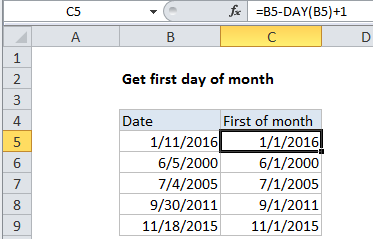## Excel Office

Excel How Tos, Tutorials, Tips & Tricks, Shortcuts

# Get first day of month in Excel

This tutorial shows how to get first day of month in Excel using the example below.

To get the first day of the month for a given date, you can use a simple formula based on the DAY function.

## Formula

`=date-DAY(date)+1`In the example shown, the formula in cell C5 is:

`=B5-DAY(B5)+1`

## Explanation

Worked Example:   How to calculate workdays per month in Excel

The DAY function returns the day value for a date. In the example show, the day value for the date in B5 (January 11, 2016) is 11. Because dates are just serial numbers in Excel, we can subtract 11, then add 1 to get the date value for January 1, 2016.

Worked Example:   Get last weekday in month in Excel

### Alternative with EOMONTH

The EOMONTH function returns the last day of in the month of a given date. This means you can get the first day of the current month with a formula like this:

`=EOMONTH(A1,-1)+1`

This formula “rolls back” a date in A1 to the last of the previous month, then adds 1. The result is the first day of the “current” month (i.e. first day of the month given by the date in A1).

Worked Example:   Sum by month in Excel